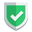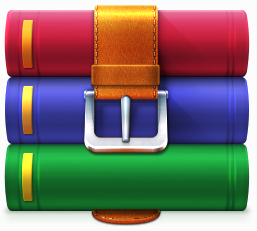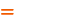我们相信：世界是美好的，你是我也是。平行空间的世界里面，不同版本的生活也在继续...

## 模型定义

``````import datetime
from django.db import models
from django.utils import timezone

class Question(models.Model):
question_text = models.CharField(max_length=200)
pub_date = models.DateTimeField('date published')
def __str__(self):
return self.question_text
def was_published_recently(self):
return self.pub_date >= timezone.now() - datetime.timedelta(days=1)

class Choice(models.Model):
choice_text = models.CharField(max_length=200)
def __str__(self):
return self.choice_text``````

## 打开`shell`界面

``python manage.py shell``

## 数据库操作（增删改查）

### 引入基本类库

``````from polls.models import Choice, Question
from django.utils import timezone``````

### 查询所有数据

``Question.objects.all()``

### 插入新数据

``````q = Question(question_text="What's new?", pub_date=timezone.now())
q.save()``````

### 查看插入的新数据的字段

``````q.id
q.question_text
q.pub_date``````

## 修改数据

``````q.question_text = "What's up?"
q.save()``````

## 普通数据查询

``Question.objects.filter(id=1)``
``Question.objects.filter(question_text__startswith='What')``
``````current_year = timezone.now().year
Question.objects.get(pub_date__year=current_year)``````
``Question.objects.get(id=2)``

## 删除数据

``q.delete()``

## 特殊数据查询

``````q = Question.objects.get(pk=1)
q.was_published_recently()``````
``````q.choice_set.all()
q.choice_set.count()``````
``````Choice.objects.filter(question__pub_date__year=current_year)
c = q.choice_set.filter(choice_text__startswith='Just hacking')``````

## 特殊数据插入

``````q.choice_set.create(choice_text='Not much', votes=0)
c = q.choice_set.create(choice_text='Just hacking again', votes=0)``````

## 总结如果本文对您有帮助，或者节约了您的时间，欢迎打赏瓶饮料，建立下友谊关系。本博客不欢迎：各种镜像采集行为。请尊重原创文章内容，转载请保留作者链接。【福利】 腾讯云最新爆款活动！1核2G云服务器首年50元！【源码】本文代码片段及相关软件，请点此获取更多信息【绝密】秘籍文章入口，仅传授于有缘之人python    django